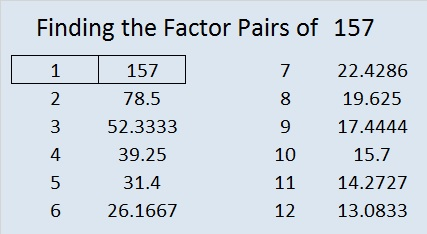# 157 and Level 3

• 157 is a prime number.
• Prime factorization: 157 is prime.
• The exponent of prime number 157 is 1. Adding 1 to that exponent we get (1 + 1) = 2. Therefore 157 has exactly 2 factors.
• Factors of 157: 1, 157
• Factor pairs: 157 = 1 x 157
• 157 has no square factors that allow its square root to be simplified. √157 ≈ 12.52996How do we know that 157 is a prime number? If 157 were not a prime number, then it would be divisible by at least one prime number less than or equal to √157 ≈ 12.5. Since 157 cannot be divided evenly by 2, 3, 5, 7, or 11, we know that 157 is a prime number.Excel file of puzzles and previous week’s factor solutions: 10 Factors 2014-06-23

A Logical Approach to FIND THE FACTORS: Find the column or row with two clues and find their common factor. Write the corresponding factors in the factor column (1st column) and factor row (top row).  Because this is a level three puzzle, you have now written a factor at the top of the factor column. Continue to work from the top of the factor column to the bottom, finding factors and filling in the factor column and the factor row one cell at a time as you go.This site uses Akismet to reduce spam. Learn how your comment data is processed.